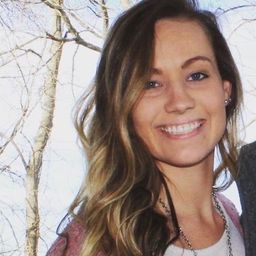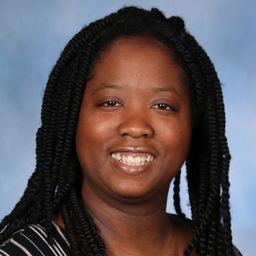Join our free STEM summer bootcamps taught by experts. Space is limited.Register Here 🏕

# California Geometry

## Educators### Problem 1

A _______ is the locus of all points in a plane that are equidistant from the endpoints of a segment. (perpendicular bisector or angle bisector).Kaci L.

### Problem 2

Use the diagram
(FIGURE CAN'T COPY)
Given that $P S=53.4, Q T=47.7,$ and $Q S=53.4$ find $P Q$Kaci L.

### Problem 3

Use the diagram
(FIGURE CAN'T COPY)
Given that $m$ is the perpendicular bisector of $\overline{P Q}$ and $S Q=25.9,$ find $S P$Kaci L.

### Problem 4

Use the diagram
(FIGURE CAN'T COPY)
Given that $m$ is the perpendicular bisector of $\overline{P Q}, P S=4 a,$ and $Q S=2 a+26,$ find $Q S$Kaci L.

### Problem 5

Use the diagram
(FIGURE CAN'T COPY)
Given that $\overrightarrow{B D}$ bisects $\angle A B C$ and $C D=21.9,$ find $A D$Kaci L.

### Problem 6

Use the diagram
(FIGURE CAN'T COPY)
Given that $A D=61, C D=61,$ and $\mathrm{m} \angle A B C=48^{\circ}$
find $\mathrm{m} \angle C B D$Kaci L.

### Problem 7

Use the diagram
(FIGURE CAN'T COPY)
Given that $D A=D C, \mathrm{m} \angle D B C=(10 y+3)^{\circ}$
and $\mathrm{m} \angle D B A=(8 y+10)^{\circ},$ find $\mathrm{m} \angle D B C$Kaci L.

### Problem 8

Carpentry For a king post truss to be constructed correctly, $P$ must lie on the bisector of $\angle J L N .$ How can braces $\overline{P K}$ and $\overline{P M}$ be used to ensure that $P$ is in the proper location?Kaci L.

### Problem 9

Write an equation in point-slope form for the perpendicular bisector of the segment with the given endpoints.
$M(-5,4), N(1,-2)$Kaci L.

### Problem 10

Write an equation in point-slope form for the perpendicular bisector of the segment with the given endpoints.
$U(2,-6), V(4,0)$Kaci L.

### Problem 11

Write an equation in point-slope form for the perpendicular bisector of the segment with the given endpoints.
$J(-7,5), K(1,-1)$Kaci L.

### Problem 12

Use the diagram
(FIGURE CANT COPY)
Given that line $t$ is the perpendicular bisector of $\overline{J K}$ and $G K=8.25,$ find $G J$Kaci L.

### Problem 13

Use the diagram
(FIGURE CANT COPY)
Given that line $t$ is the perpendicular bisector If $\overline{I K}, I G=x+12,$ and $K G=3 x-17,$ find $K G$Kaci L.

### Problem 14

Use the diagram
(FIGURE CANT COPY)
Given that $G=70.2, J H=26.5,$ and $G K=70.2,$ find $J K$Kaci L.

### Problem 15

Use the diagram
(FIGURE CANT COPY)
Given that $\mathrm{m} \angle R S Q=\mathrm{m} \angle T S Q$ and $T Q=1.3,$ find $R Q$Kaci L.

### Problem 16

Use the diagram
(FIGURE CANT COPY)
Given that $\mathrm{m} \angle R S Q=58^{\circ}, R Q=49,$ and $T Q=49$
find $\mathrm{m} \angle R S T$Kaci L.

### Problem 17

Use the diagram
(FIGURE CANT COPY)
Given that $R Q=T Q, \mathrm{m} \angle Q S R=(9 a+48)^{\circ}$
and $\mathrm{m} \angle Q S T=(6 a+50)^{\circ},$ find $\mathrm{m} \angle Q S T$Kaci L.

### Problem 18

The planners for a new section of the city want every location on Main Street to be equidistant from Elm Street and Grove Street. How can the planners ensure that this is the case?Kaci L.

### Problem 19

Write an equation in point-slope form for the perpendicular bisector of the segment with the given endpoints.
$E(-4,-7), F(0,1)$Kaci L.

### Problem 20

Write an equation in point-slope form for the perpendicular bisector of the segment with the given endpoints.
$X(-7,5), Y(-1,-1)$Kaci L.

### Problem 21

Write an equation in point-slope form for the perpendicular bisector of the segment with the given endpoints.
$$M(-3,-1), N(7,-5)$$Kaci L.

### Problem 22

Write an equation in point-slope form for the perpendicular bisector of the segment with the given endpoints.
$\overline{P Q}$ is the perpendicular bisector of $\overline{S T}$ Find the values of $m$ and $n .$
(FIGURE CAN'T COPY)Ashley H.

### Problem 23

Use the diagram of a shuffleboard and the following information to find each length.
$\overline{K Z}$ is the perpendicular bisector of $\overline{G N}, \overline{H M},$ and $\overline{J L}$
$$J K$$Ashley H.

### Problem 24

Use the diagram of a shuffleboard and the following information to find each length.
$\overline{K Z}$ is the perpendicular bisector of $\overline{G N}, \overline{H M},$ and $\overline{J L}$.
$$G N$$Ashley H.

### Problem 25

Use the diagram of a shuffleboard and the following information to find each length.
$\overline{K Z}$ is the perpendicular bisector of $\overline{G N}, \overline{H M},$ and $\overline{J L}$.$$M L$$Ashley H.

### Problem 26

Use the diagram of a shuffleboard and the following information to find each length.
$\overline{K Z}$ is the perpendicular bisector of $\overline{G N}, \overline{H M},$ and $\overline{J L}$
$$H Y$$Ashley H.

### Problem 27

Use the diagram of a shuffleboard and the following information to find each length.
$\overline{K Z}$ is the perpendicular bisector of $\overline{G N}, \overline{H M},$ and $\overline{J L}$
$$J L$$Ashley H.

### Problem 28

Use the diagram of a shuffleboard and the following information to find each length.
$\overline{K Z}$ is the perpendicular bisector of $\overline{G N}, \overline{H M},$ and $\overline{J L}$$N M$$Ashley H. Numerade Educator ### Problem 29 The endpoints of$\overline{A B}$are$A(-2,1)$and$B(4,-3) .$Find the coordinates of a point$C$other than the midpoint of$\overline{A B}$that is on the perpendicular bisector of$\overline{A B} .$How do you know it is on the perpendicular bisector?Ashley H. Numerade Educator ### Problem 30 Write a paragraph proof of the Converse of the Perpendicular Bisector Theorem. Given:$A X=B X$Prove:$X$is on the perpendicular bisector of$\overline{A B}$. Plan: Draw$\ell$perpendicular to$\overline{A B}$through$X .$Show that$\triangle A Y X \cong \triangle B Y X$and thus$\overline{A Y} \cong \overline{B Y} .$By definition,$\ell$is the perpendicular bisector of$\overline{A B}$. (FIGURE CANT COPY)Ashley H. Numerade Educator ### Problem 31 Write a two-column proof of the Angle Bisector Theorem. Given:$\overrightarrow{P S}$bisects$\angle Q P R . \overline{S Q} \perp \overrightarrow{P Q}, \overline{S R} \perp \overrightarrow{P R}$Prove:$S Q=S R$Plan: Use the definitions of angle bisector and perpendicular to identify two pairs of congruent angles. Show that$\triangle P Q S \cong \triangle P R S$and thus$\overline{S Q} \cong \overline{S R}$Ashley H. Numerade Educator ### Problem 32 In the Converse of the Angle Bisector Theorem, why is it important to say that the point must be in the interior of the angle?Ashley H. Numerade Educator ### Problem 33 This problem will prepare you for the Concept Connection on page 328 . A music company has stores in Abby$(-3,-2)$and Cardenas$(3,6)$Each unit in the coordinate plane represents 1 mile. a. The company president wants to build a warehouse that is equidistant from the two stores. Write an equation that describes the possible locations. b. A straight road connects Abby and Cardenas. The warehouse will be located exactly 4 miles from the road. How many locations are possible? c. To the nearest tenth of a mile, how far will the warehouse be from each store?Ashley H. Numerade Educator ### Problem 34 How is the construction of the perpendicular bisector of a segment related to the Converse of the Perpendicular Bisector Theorem?Ashley H. Numerade Educator ### Problem 35 If$\overrightarrow{J K}$is perpendicular to$\overline{X Y}$at its midpoint$M,$which statement is true? A$J X=K Y$B$J X=K X$C$J M=K M$D$J X=J Y$Ashley H. Numerade Educator ### Problem 36 What information is needed to conclude that$\overrightarrow{E F}$is the bisector of$\angle D E G ?$C$\mathrm{m} \angle D E F=\mathrm{m} \angle D E G$G)$\mathrm{m} \angle G E D=\mathrm{m} \angle G E F$G$\mathrm{m} \angle F E G=\mathrm{m} \angle D E F\odot \mathrm{m} \angle D E F=\mathrm{m} \angle E F G$Ashley H. Numerade Educator ### Problem 37 The city wants to build a visitor center in the park so that it is equidistant from Park Street and Washington Avenue. They also want the visitor center to be equidistant from the museum and the library. Find the point$V$where the visitor center should be built. Explain your answer.Ashley H. Numerade Educator ### Problem 38 Consider the points$P(2,0), A(-4,2), B(0,-6),$and$C(6,-3)$a. Show that$P$is on the bisector of$\angle A B C$. b. Write an equation of the line that contains the bisector of$\angle A B C$.Ashley H. Numerade Educator ### Problem 39 Eind the locus of points that are equidistant from the$x$-axis and$y$-axis.Ashley H. Numerade Educator ### Problem 40 Write a two-column proof of the Converse of the Angle Bisector Theorem. Given:$\overline{V X} \perp \overrightarrow{Y X}, \overline{V Z} \perp \overrightarrow{Y Z}, V X=V Z$Prove:$\overrightarrow{Y V}$bisects$\angle X Y Z$(FIGURE CANT COPY)Ashley H. Numerade Educator ### Problem 41 Write a paragraph proof. Given:$\overline{K N}$is the perpendicular bisector of$\overline{I L}\overline{L N}$is the perpendicular bisector of$\overline{K M}\overline{J R} \cong \overline{M T}$Prove:$\angle J K M \cong \angle M L J$(FIGURE CANT COPY)Ashley H. Numerade Educator ### Problem 42 Lyn bought a sweater for$\$16.95 .$ The change $c$ that she received can be described by $c=t-16.95,$ where $t$ is the amount of money Lyn gave the cashier. What is the dependent variable? (Previous course)Ashley H.

### Problem 43

For the points $R(-4,2), S(1,4), T(3,-1),$ and $V(-7,-5),$ determine whether the Infin ars narallsh nernendiswlar, ar neither.
$\overleftarrow{R S}$ and $\overleftarrow{V T}$Ashley H.

### Problem 44

For the points $R(-4,2), S(1,4), T(3,-1),$ and $V(-7,-5),$ determine whether the Infin ars narallsh $\overleftarrow{R V}$ and $\overleftarrow{S T}$Ashley H.

### Problem 45

For the points $R(-4,2), S(1,4), T(3,-1),$ and $V(-7,-5),$ determine whether the Infin ars narallsh nernendiswlar, ar neither.
$\overrightarrow{R T}$ and $\overrightarrow{V R}$Ashley H.

### Problem 46

Write the equation of each line in slope-intercept form
the line through the points $(1,-1)$ and $(2,-9)$Ashley H.
The line with $x$ -intercept $-4$ and $y$ -intercept 5